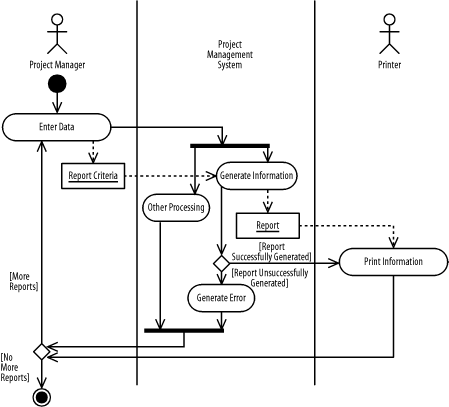### B.2 Behavioral Modeling

The next few sections present solutions for Part III.

#### B.2.1 Chapter 6

1. Figure B-32 shows the sequence diagram, and Figure B-33 shows the collaboration diagram. The interaction and collaboration is that of the Generate Project-Status Report described in Chapter 6.

In Figure B-32, the following elements are missing:

• ELM-01 should be:

`4 : OutputData := FormatInfo (OrgInfo, PrjInfo)`
• ELM-02 should be:

`12 : OutputData := FormatWorkerInfo (UnitsOfWork, WorkProducts)`
• ELM-03 should be:

`[No more workers]`
##### Figure B-32. Sequence diagram (question 1)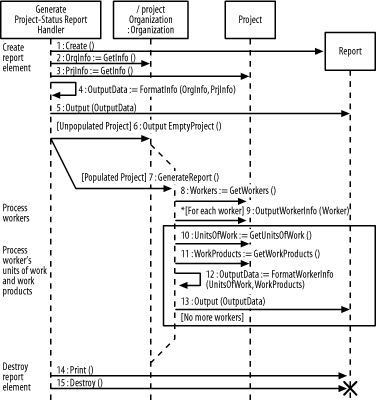In Figure B-33, the following elements are missing:

• ELM-04 should be:

`[Populated Project] 7 : GenerateReport(  )`
• ELM-05 should be:

`*[For each worker] 7.2 : outputWorkerInfo (Worker)`
• ELM-06 should be:

`9 : Destroy (  )`
##### Figure B-33. Collaboration diagram (question 1)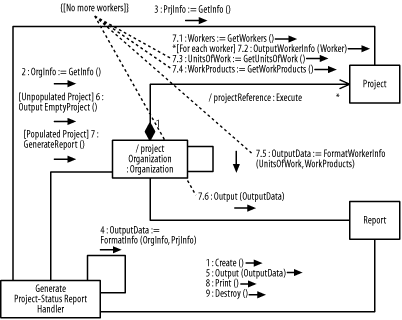2. The following describes the figure: there are three elements, named Section, Report, and OutputDeviceInterface. The Report element has a Use relationship with the OutputDeviceInterface element and a Has relationship with the Section element.

Because these figures show an interaction and collaboration, each step adds to the previous step; otherwise, the notion of when a communication occurs would be lost!

1. Figure B-34 and Figure B-35 show the figures.

##### Figure B-34. Sequence diagram (question 2 part a)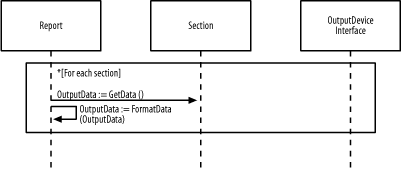##### Figure B-35. Collaboration diagram (question 2 part a)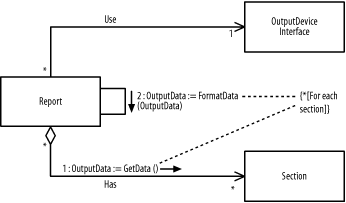2. Figure B-36 and Figure B-37 show the figures.

##### Figure B-36. Sequence diagram (question 2 part b)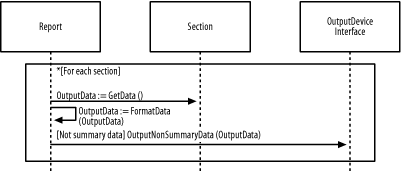##### Figure B-37. Collaboration diagram (question 2 part b)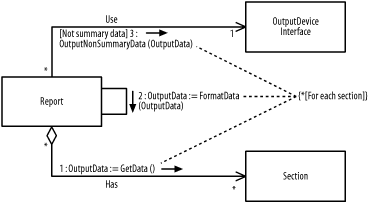3. Figure B-38 and Figure B-39 show the figures.

##### Figure B-38. Sequence diagram (question 2 part c)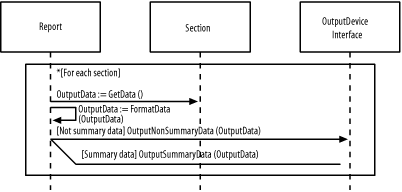##### Figure B-39. Collaboration diagram (question 2 part c)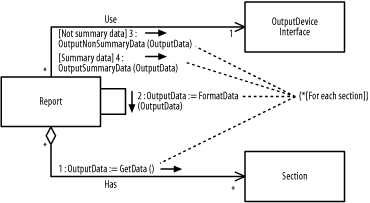#### B.2.2 Chapter 7

1. The following describes the figure:

• The Minimized state indicates that a window is displayed as an icon taking a minimal amount of space on the user screen.

• The Restored state indicates that a window is displayed as one of many windows taking a portion of the space on the user screen.

• The Maximized state indicates that a window is displayed as the only window taking all the space on the user screen.

• When a window is created using the Open event or operation, it enters the Restored state.

• When a window is destroyed using the Close event or operation, it may be in any simple state, including the Minimized, Restored, or Maximized state.

2. The following update the figure:

1. Figure B-40 shows the figure.

##### Figure B-40. State diagram (question 2 part a)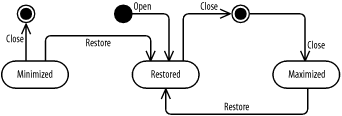2. Figure B-41 shows the figure.

##### Figure B-41. State diagram (question 2 part b)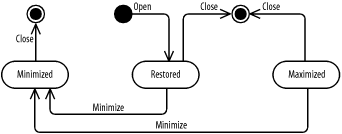3. Figure B-42 shows the figure.

##### Figure B-42. State diagram (question 2 part c)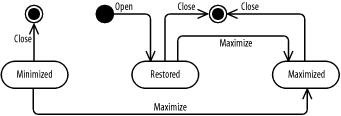4. Figure B-43 shows the figure.

##### Figure B-43. State diagram (question 2 part d)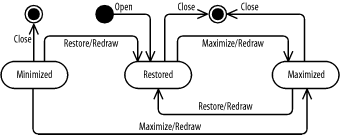5. Figure B-44 shows the figure.

##### Figure B-44. State diagram (question 2 part e)Figure B-45 shows the resulting diagram, which includes all the steps in this question.

##### Figure B-45. State diagram (question 2)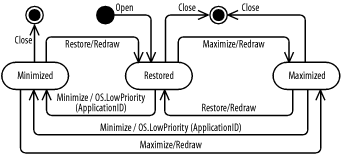#### B.2.3 Chapter 8

1. The following describes the figure:

• The Project Manager swimlane shows the action states that are the responsibility of a project manager.

• The Project Management System swimlane shows the action states that are the responsibility of the project management system.

• The Printer swimlane shows the action states that are the responsibility of a printer.

• First, the project manager enters data using the Enter Data action state. Next, the project management system generates the report using the Generate Information action state. Finally, the printer prints the report using the Print Information action state.

• The Generate Information action state outputs a Report object and the Print Information action state inputs the Report object.

• After the printer prints a report using the Print Information action state, a project manager may choose to print more than one report.

2. The following update the figure:

1. Figure B-46 shows the figure.

##### Figure B-46. Activity diagram (question 2 part a)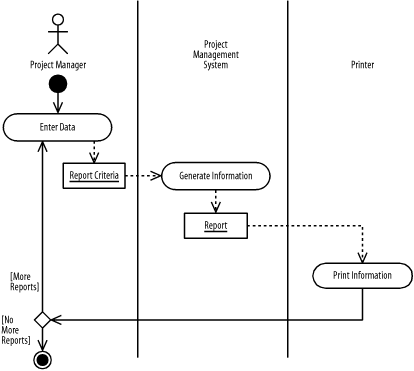2. Figure B-47 shows the figure.

##### Figure B-47. Activity diagram (question 2 part b)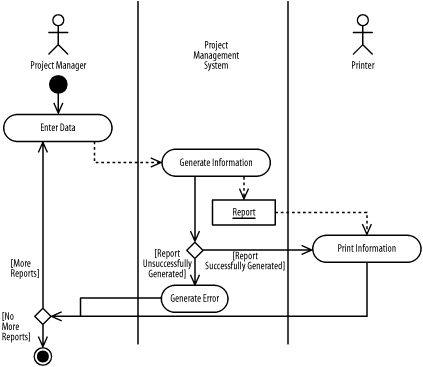3. Figure B-48 shows the figure.

##### Figure B-48. Activity diagram (question 2 part c)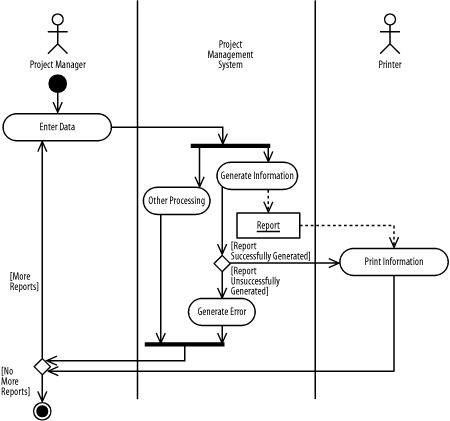Figure B-49 shows the resulting diagram, which includes all the steps in this question.

##### Figure B-49. Activity diagram (question 2)